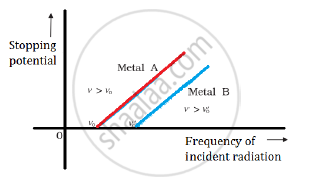Share

# Define Stopping Potential and Threshold Frequency, Using Einstein’S Equation and Drawing Necessary Plot Between Relevant Quantities. - CBSE (Science) Class 12 - Physics

ConceptEinstein’s Equation - Particle Nature of Light

#### Question

Define (i) stopping potential and (ii) threshold frequency, using Einstein’s equation and drawing necessary plot between relevant quantities.

#### Solution

Stopping potential: For a particular frequency of incident radiation, the minimum negative (retarding) potential V0 for which the photocurrent stops or becomes zero is called the cut-off or stopping potential.

Threshold frequency: The minimum frequency required for photoelectrons to be emitted from a metal surface is called the threshold frequency.

The graph between stopping potential and frequency of incident radiation is shown below:Is there an error in this question or solution?

#### APPEARS IN

Solution Define Stopping Potential and Threshold Frequency, Using Einstein’S Equation and Drawing Necessary Plot Between Relevant Quantities. Concept: Einstein’s Equation - Particle Nature of Light.
S